3.8 Elapsed Time

# Learning Objectives

• Add and subtract time.
• Solve elapsed time questions using a variety of methods.

# Introduction

Beating the Clock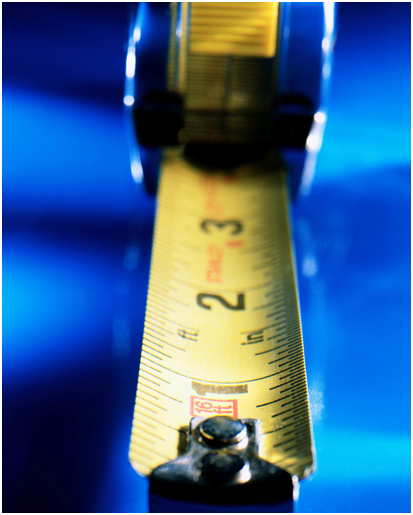While working, Travis accidentally loses his measuring tape. He searches everywhere, but can’t seem to find it.

“Uncle Larry, I can’t find my measuring tape,” Travis says. “I’m going to ride my bike to the hardware store and get a new one.”

“Alright Travis, but we are having a meeting at 11 and I would like you to be back for it,” Uncle Larry says.

Travis looks down at his watch. It is 10:15. He knows that he can make it, but he will need to hurry.

Travis dashes out the door and jumps onto his bike. He rides to the store, gets his measuring tape and returns back.

It takes Travishour to ride to the store and back. He gets a little distracted in the store, but after 10 minutes, he finds and buys the measuring tape.

Did Travis make it back in time for the meeting? You will need to learn some information about calculating time to be sure.

Pay close attention and you will be able to figure this problem out at the end of the lesson.

# Guided Learning

## Add and Subtract Measures of Time

We add and subtract units of time every day. Sometimes, we are trying to figure out whether we will be on time or late. In other situations, we are trying to figure out a movie time or the time to meet a friend, or how long the soccer game actually lasted. To calculate units of time, we are going to need to know how to convert minutes to seconds to hours.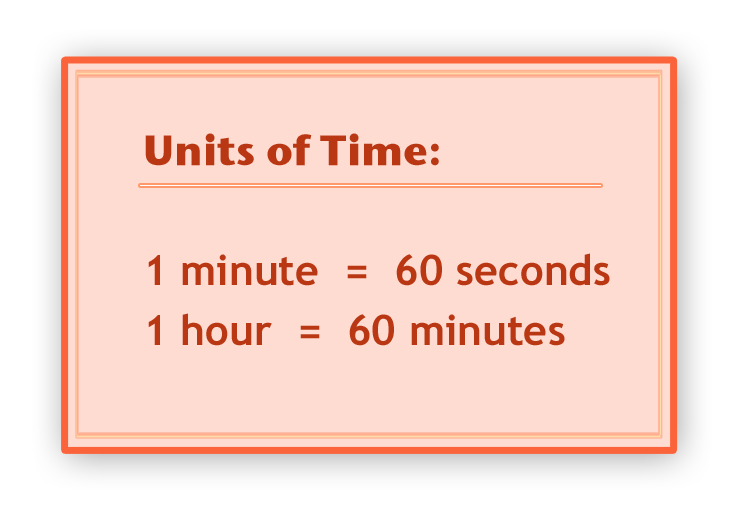One of the first things that you need to know how to do when working with time is to convert different units.

How do we convert units?

The easiest way is to simply multiply or divide. Sometimes, you will be able to accomplish this using mental math. In fact, you should try to figure things out in your head whenever possible. In this lesson, you can see the work the long way too, but mental math is almost always quicker.

### Example A

120 minutes = _______ hours

First, try to figure this one out in your head using mental math. Now let’s look at the solution.

To convert a smaller unit to a larger unit, we divide.

There are 60 minutes in one hour, so we divide 120 minutes by 60 and we get two.

120 minutes = 2 hours

We can also do this the other way around.

### Example B

How many minutes are there in four hours?

To solve this problem, we are going to from a larger unit to a smaller unit, so we multiply.

4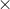60240

There are 240 minutes in four hours.

## Guided Practice

Try a few of these on your own.

1. 180 minutes = ______ hours
2. 5 hours = ______ minutes
3. 180 seconds = ______ minutes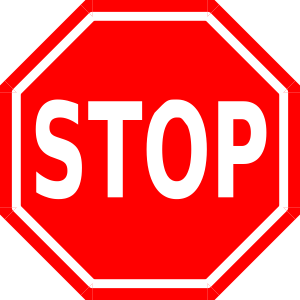Solutions:

1. 3 hours
2. 300 minutes
3. 3 minutes

What about when you have fractional units of time?

Sometimes, we measure time using fractional units. For example, we might use the phraseof an hour oran hour. We can also figure out how many minutes these fractional units of time are.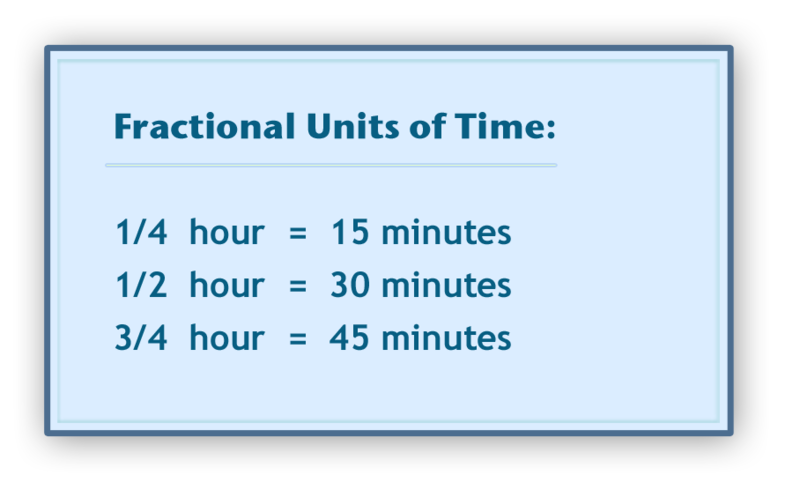Here are some given fractional units of time. We can figure out whether or not these measures are accurate.

Let’s test out and prove thathour is equal to 15 minutes. If we know that there are 60 minutes in one hour then we can multiply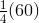and that will give us the number of minutes.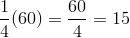Our work is accurate.

We can also figure out any fraction of an hour using this method.

### Example C

What isof an hour in minutes?

To figure this out, we multiplytimes 60 since we want our answer in minutes.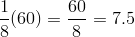Our answer is 7.5 minutes orminutes.

## Guided Practice

Try a few of these conversions on your own.

1. How many minutes isof an hour?
2. How many minutes isof an hour?Take a few minutes to check your work with a partner.

Solutions:

1. 12 minutes
2. 10 minutes

## Find Elapsed Time Between Given Start and Stop Times

Elapsed time tells us how much time passes between two events. We can calculate the elapsed time by figuring out the difference between a start and a stop time.

### Example D

Soccer practice begins at 3:15 pm and ends at 4:45 pm. Determine how long soccer practice lasts.

To solve this problem, we need to set up a subtraction problem that could be used to find the number of hours and minutes that pass between those two times. Subtract the starting time from the stopping time. Each time in the problem above represents the number of minutes and hours past noon.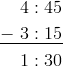Soccer practice lasts for 1 hour 30 minutes.

Sometimes, we will need to rename the times in a problem in order to subtract them.

### Example E

A dance performance starts at 7:30 pm and ends at 10:10 pm. How long does the performance last?

Subtract to find the amount of time that passes between those two times.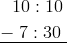You cannot subtract 30 minutes from 10 minutes, so you must rewrite 10:10.

Remember, 10:10 stands for 10 hours and 10 minutes past noon.

Rewrite that time as follows. Remember that 1 hour = 60 minutes.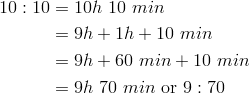Rewrite 10:10 as 9:70. Then subtract.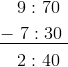The dance performance lasts for 2 hours 40 minutes.

What about when an event starts in the morning and ends in the afternoon, how do we calculate elapsed time then?

To do this, we are going to need a different strategy. Let’s look at an example.

### Example F

The soccer game started at 10:00 am and ended at 1:40 pm. How long was the game?

To figure this out, we must first count up to noon. From 10 to Noon is 2 hours, so we need to keep track of that time.

2 hours.

Then we can figure out how long it was from noon to 1:40 pm. That is 1 hour and 40 minutes.

Finally, we can add the two figures together.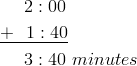## Guided Practice

Try a few of these on your own.

1. The game started at 9:10 am and ended at 11:15 am. What was the length of the game?
2. The movie started at 7:30 pm and ended at 10:20 pm. How long was the movie?Check your work with a peer.

Solutions:

1. 2 hours and 5 minutes
2. 2 hours and 50 minutes

# Real Life Example Completed

Beating the ClockYou have learned all about how to calculate units of time and elapsed time. Now let’s go back to our original problem.

While working, Travis accidentally loses his measuring tape. He searches everywhere, but can’t seem to find it.

“Uncle Larry, I can’t find my measuring tape,” Travis says. “I’m going to ride my bike to the hardware store and get a new one.”

“Alright Travis, but we are having a meeting at 11 and I would like you to be back for it,” Uncle Larry says.

Travis looks down at his watch. It is 10:15. He knows that he can make it, but he will need to hurry.

Travis dashes out the door and jumps onto his bike. He rides to the store, gets his measuring tape and returns back.

It takes Travishour to ride to the store and back. He gets a little distracted in the store, but after 10 minutes, he finds and buys the measuring tape.

Did Travis make it back in time for the meeting? You will need to learn some information about calculating time to be sure.

First, let’s go back and underline all of the important information.

Now we need to add up the time that it took Travis to go to the store, buy the measuring tape and get back.

Riding time = thirty minutes of riding time total; 15 minutes there and 15 minutes back.

Ten minutes in the store.

30 + 10 = 40 total minutes

How much time was there from 10:15 when Travis left until the meeting at 11:00?

11 - 10:15 = 45 minutes

45 - 40 = 5 minutes

Travis arrived back at the work site with five minutes to spare.

# Review

• A numerical expression is an expression with multiple numbers and multiple operations.

Elapsed time

How much time passes between two events.

# Video Resources

Elapsed Time Video

Operations with Time Video